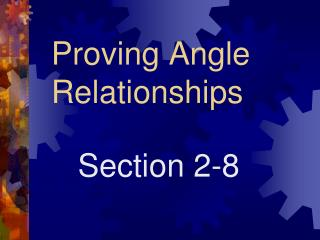Download PresentationProving Angle Relationships

Proving Angle Relationships - PowerPoint PPT Presentation

Proving Angle Relationships. Section 2-8. Protractor Postulate Given and a number r between 0 and 180, there is exactly one ray with endpoint A, extending on either side of , such that the measure of the angle formed is r. Angle Addition Postulate.I am the owner, or an agent authorized to act on behalf of the owner, of the copyrighted work described.
Download PresentationProving Angle Relationships

Download Policy: Content on the Website is provided to you AS IS for your information and personal use and may not be sold / licensed / shared on other websites without getting consent from its author.While downloading, if for some reason you are not able to download a presentation, the publisher may have deleted the file from their server.

- - - - - - - - - - - - - - - - - - - - - - - - - - E N D - - - - - - - - - - - - - - - - - - - - - - - - - -
Presentation Transcript
1. Proving Angle Relationships Section 2-8

2. Protractor Postulate Given and a number r between 0 and 180, there is exactly one ray with endpoint A, extending on either side of , such that the measure of the angle formed is r.

3. Angle Addition Postulate If R is in the interior of , then Converse is also true. P R Q S

4. Supplement Theorem (2.3) • If 2 angle form a linear pair, then they are supplementary angles. 120 60

5. Complement Theorem (2.4) • If the noncommon sides of 2 adjacent angles form a right angle, then the angles are complementary angles.

6. Theorem 2-5 • Congruence of angles is reflexive, symmetric, and transitive.

7. Theorems 2-6 and 2-7 • Angles supplementary to the same angle or to congruent angles are congruent. • Angles complementary to the same angle or to congruent angles are congruent.

8. Theorems 2.8 - 2.13 • All right angles are congruent.(2.10) • Vertical angles are congruent.(2.8) • Perpendicular lines intersect to form 4 right angles. (2.9) 140 40 40 140

9. Perpendicular lines form congruent adjacent angles. (2.11) • If 2 angles are congruent and supplementary, then each angle is a right angle. (2.12) • If 2 congruent angles form a linear pair, then they are right angles.

10. Joke Time Why do bees have sticky hair? Because they have honeycombs!

11. What goes Oh, Oh, Oh? Santa walking backwards.